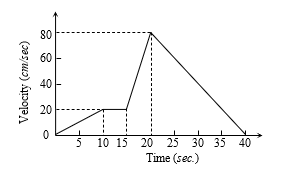Average Acceleration
Question

# The v-t  graph of a moving object is given in figure. The maximum acceleration isEasy
Solution

## Maximum acceleration means maximum change in velocity in minimum time interval. In time interval$\mathrm{a}=\frac{\mathrm{\Delta v}}{\mathrm{\Delta t}}=\frac{80-20}{20-15}=\frac{60}{5}=12\text{ }\mathrm{cm}/{\mathrm{sec}}^{2}$

Get Instant Solutions